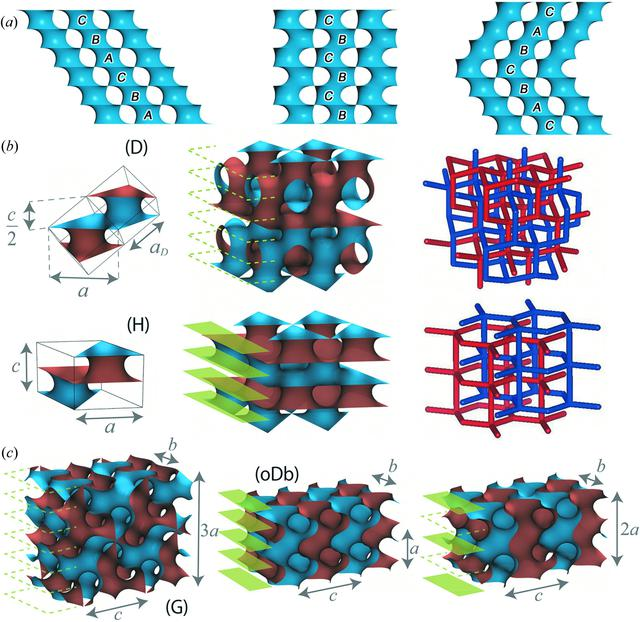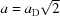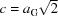disable zoom     view article Figure 5 Structural variations associated with D and G twins. (a) Possible stacking orders of catenoidal necks. Each catenoidal neck is spanned by two equilateral triangles having a common threefold axis, where the triangles are parallel or anti-parallel if the arrangements of necks in the two adjacent layers are the same (as in the H surfaces) or different (as in the D surface), respectively. For D twins, necks with parallel triangles exist only at the twin boundary. (b) The D surface (1st row) and an H surface (2nd row) shown along with their smallest unit cells and labyrinth networks. Reference unit-cell constants are taken from the cubic D surface, such thatand c/a = (2/3)1/2 where aD is the cubic lattice constant. (c) The G surface (left) and the first (middle) and second (right) simplest polysynthetic G twins. The reference unit-cell constants are taken from the cubic G surface, such that, a/c = (1/3)1/2 and b/c = (3/8)1/2 where aG is the cubic lattice constant. In (b) and (c) all the surfaces are oriented with sides being coloured blue and brown. The dashed green lines indicate the candidate positions of twin boundaries (i.e. {111} + 0.5 and {211} + 0.5, respectively), while the green plates indicate actual reflection planes. The periods along orthogonal axes are shown with double-sided arrows and their lengths.

IUCrJ
Volume 7| Part 2| March 2020| Pages 228-237
ISSN: 2052-2525